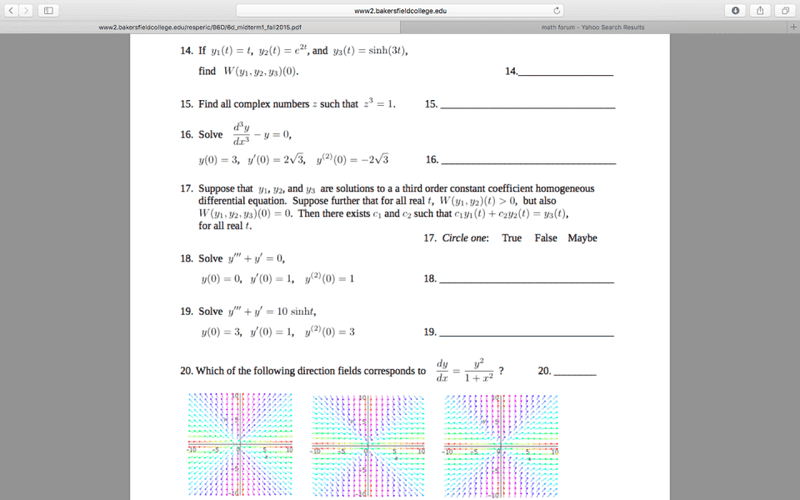# Ordinary Differential equations question

• Hutchyy

#### Hutchyy

#17 If you can't see the picture: Suppose that y1, y2, and y3 are solutions to a third order constant coefficient homogeneous differential equation. Suppose further that for all real t, W(y1,y2)(t)>0, but also W(y1,y2,y3)(0)=0. Then there exists c1 and c2 such that c1y1(t) + c2y2(t) =y3(t) for all real t. Is this true, false or maybe true? I know it must have something to do with abel’s theorem but I can’t really figure out how it applies.. ,

what is the significance of plugging in 0 for ’t’ in the wronskian?

I'm thinking its something that has to do with abel's theorem but I can't make any connections as to how having a positive wronskian relates to a bigger wronskian with 0 plugged into equalling 0. https://www.physicsforums.com/attachments/90663

There's something wrong with your attachment. I can't view it.When y= 0 (along the x-axis), dy/dx= 0. Which of the direction fields has that property? When x= 0 (along the y axis) dy/dx= y^2. Which of the direction fields has that property?

Thanks halls of ivy! But I got that one! I meant number 17 the word problem